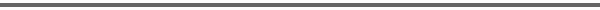Journal Home Page Complete Contentsof this Volume Previous Article Next Article Journal of Lie Theory 14 (2004), No. 2, 339--370 Copyright Heldermann Verlag 2004Classification of Spherical Nilpotent Orbits in Complex Symmetric Space Donald R. King Dept. of Mathematics, Northeastern University, 567 Lake Hall, Boston MA 02115, U.S.A., donking@neu.edu[Abstract-pdf] Let $G$ be the adjoint group of the simple real Lie algebra $\g$, and let $K_{{}_{\bf C}}~\rightarrow~{{\rm Aut}({\frak p}_{{}_{\bf C}})}$ be the complexified isotropy representation at the identity coset of the corresponding symmetric space. We classify the spherical nilpotent $K_{{}_{\bf C}}$ orbits in ${\frak p}_{{}_{\bf C}}$. [FullText-pdf (282 KB)] for subscribers only.